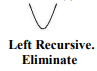Courses

# Left Factoring and Examples - Compiler Design, CSE & IT Engineering | EduRev Notes

## Computer Science Engineering (CSE) : Left Factoring and Examples - Compiler Design, CSE & IT Engineering | EduRev Notes

The document Left Factoring and Examples - Compiler Design, CSE & IT Engineering | EduRev Notes is a part of the Computer Science Engineering (CSE) Course Compiler Design.
All you need of Computer Science Engineering (CSE) at this link: Computer Science Engineering (CSE)

• Example 1: Remove the left recursion from the production: A ⇒ A α|βApplying the transformation yields:

A→ β A I
A I→ α A I | ∈
­­­
RemAIning part after A

Example 2:
Remove the left recursion from the productions:
E → E + T | T
T→ T * F | F
Applying the transformation yields:
E→ T EI                                                  T → F TI
E I→ T EI | ∈                                        T I→ * F TI

Example 3:
Remove the left recursion from the productions:
E→ E + T | E – T|T
T→ T * F| T/F | F
Applying the transformation yields:
E→ T E                          T→ F TI
E→ + T EI | - T EI | ∈       T I→ * F T| /F TI | ∈

Example 4:
Remove the left recursion from the productions:
S→ A a | b
A→ A c | S d | ∈
1. The non terminal S is left recursive because S→ A a→ S d a But it is not immediate left recursive.
2. Substitute S-productions in A→ S d to obtAIn:
A→ A c | A a d | b d | ∈
3. Eliminating the immediate left recursion:
S→ A a | b
A→ b d AI | A
AI→ c AI | a d A|∈

Example 5:
Consider the following grammar and eliminate left recursion. S → A a | b
A → S c | d

The nonterminal S is left recursive in two steps: S → A a → S c a → A a c a → S c a c a - - -

Left recursion causes the parser to loop like this, so remove: Replace A → S c | d by A → A a c | b c | d

and then by using Transformation rules: A → b c AI | d A
AI → a c AI

2.5 Left Factoring: Left factoring is a grammar transformation that is useful for producing a grammar suitable for predictive parsing.
When it is not clear which of two alternative productions to use to expand a non-terminal A, we may be able to rewrite the productions to defer the decision until we have some enough of the input to make the right choice.

Algorithm: For all A ∈ non-terminal, find the longest prefix a that occurs in two or more right-hand sides of A.

If a ¹ ∈ then replace all of the A productions, A → a bI | a b2 | - - - | a bn | r
With
A → a AI | r AI → bI | b2| - - - | bn | ∈ Where, AI is a new element of non-terminal. Repeat until no common prefixes remAIn.
It is easy to remove common prefixes by left factoring, creating new non-terminal.

For example consider:
V → a b | a r Change to:
V → a VI V→ b | r

Example 1:
Eliminate Left factoring in the grammar: S → V := int
V → alpha ‘[‘ int ’]’ | alpha
Becomes:
S → V := int
V → alpha VI
VI → ’[‘ int ’] | Î

Offer running on EduRev: Apply code STAYHOME200 to get INR 200 off on our premium plan EduRev Infinity!

## Compiler Design

16 videos|44 docs|29 tests

,

,

,

,

,

,

,

,

,

,

,

,

,

,

,

,

,

,

,

,

,

,

,

,

;